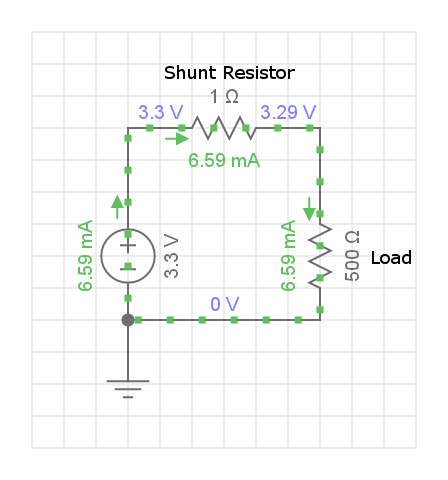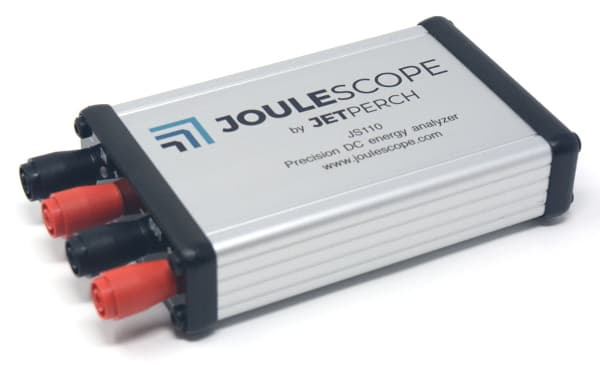# How to measure current

Electrical current is the flow of electric charge from positive voltage to negative voltage. We now know that electrons move through conductors from negative voltages to positive voltage. We had a 5050 chance of guessing “right”, so, yeah.

Electrical current is the flow of charge per time, which is measured in units of ampere (A), often called an “amp”. One amp is one coloumb of charge flowing per second. One coloumb is approximately 6.242 x 1018 electrons!

A device that measures current is called an ammeter.

## Primary Techniques

Directly measuring current is difficult. Counting individual electrons is not usually an option for electric circuits. Therefore, the two primary techniques use “side-effects” of current. First, moving charged particles create a magnetic field (Ampère’s Law), and charged particles moving through resistance create a voltage (Ohm’s Law). Both of these techniques can now be derived from Maxwell’s equations.

### Magnetic field

Current creates a magnetic field that was first discovered by Ørsted in 1820 using a compass. This technique was later refined into the modern galvanometer.

Most modern galvanometers have a permanent magnet and a coil of wire. When current flows through the coil, it pushes towards or away from the permanent magnet. The coil is attached to a needle and torsion spring. If you have ever seen analog multimeters or vintage stereo equipment, you have likely seen a galvanometer.

The magnetic field can also be measured using hall-effect sensors. Changing magnetic fields (AC) can use a sense coil through inductance.

As the current gets smaller (under 1 mA), measuring the magnetic field with sufficient precision becomes difficult.

### Shunt resistors

When a resistor is placed in the path of current, it produces a voltage according to Ohms’s Law:

``````V = I * R
``````

Solving for current:

``````I = V / R
``````

If the resistance is known and we measure voltage, we can compute current. Most modern ammeters use shunt resistors. The best part about this approach is that we can select a shunt resistor value that gives us a suitable voltage range!A shunt resistor is also called a “current sense resistor”, or simply “sense resistor”.

By design, shunt resistors cause a voltage drop, also called burden voltage or insertion loss. If this voltage is too large, it affects the load. The additional resistance also changes the source impedance as seen by the load, which can cause some load circuits to behave differently. Ideally, the shunt resistance would be so small that it would not affect the target circuit. Practically, the shunt resistance has to create a measurable voltage.

Measuring a large current range is difficult for a single shunt resistor. The voltmeter has a fixed range. To expand the range, most ammeters use multiple shunt resistors, each with different resistances. However, if the current changes over time, a shunt resistor that is too large can cause an excessive voltage drop that affects the behavior of the target circuit. If the shunt resistor is too small, it cannot accurately measure the current.

## Equipment

### Multimeters

Most multimeters can measure current. These multimeters have internal shunt resistors, and they actually measure voltage! When you change multimeter ranges, you are selecting different shunt resistors, usually with two different voltage gain settings.

Multimeters are well-suited for measuring currents that are constant, either as direct current or “constant” RMS alternating current. Multimeters cannot easily measure currents that vary rapidly or that change dramatically over time.

Some multimeters also support current clamps. These clamps fit over wires to measure the current through the emitted magnetic field. DC current clamps often have a hall-effect sensor. AC current clamps often use magnetic coils. Some current clamps support both DC and AC measurements. Current clamps are practically limited to resolutions around 1 mA, which is not sufficient for many applications.

### Oscilloscopes

Oscilloscopes measure voltages at regular intervals, often over a million times per second, to construct a voltage waveform. Oscilloscopes then display a graph showing changes in voltage over time. By measuring the voltage over an external shunt resistor, oscilloscopes can effectively display changes in current over time.

However, this approach has two primary challenges. First, the shunt resistor measurement technique has the dynamic range issues associated with shunt resistors. Oscilloscopes usually trade-off speed for limited dynamic range, and typically have just 10 or 12 bits of dynamic range.

Second, oscilloscopes are usually earth ground referenced. The oscilloscope measures the voltage difference between earth ground and the signal. However, we want the differential measurement across the shunt resistor. Introducing shunt resistance into the ground path often causes signal integrity issues. We often want “high-side” shunt resistors on the positive power supply. However, if the test circuit is also earth ground referenced, we cannot use the oscilloscope’s standard probe to measure the voltage difference across the shunt resistors. We can either use two oscilloscope probes and use a mathematical subtract feature, which introduces additional measurement error, or we can use a differential oscilloscope probe, which are often quite expensive. Either way, we are still left with the dynamic range issue.

Oscilloscope manufacturers also provide current probes, which are usually just a combined shunt resistor and differential probe. These probes also allow the oscilloscope to get the units right so that you don’t have to do Ohm’s law calculations every time you measure current. However, you still have limited dynamic range.

Oscilloscopes also have current clamp probes, which are limited to around 1 mA resolution.

### Specialized Equipment

A variety of other equipment exists that can measure current, sometimes while sourcing or sinking current. Equipment of this type includes electrometers, picoammeters, and Source Measurement Units (SMUs). These products are specially designed to overcome some of the standard multimeter drawbacks, and many do have lower burden voltages and lower input bias currents. However, the primary drawback is cost. These devices often employ multiple, more complicated, active feedback ammeter sensing methods.

How do we overcome these limitations without breaking the bank? What if we had a device that automatically and instantaneously selected the shunt resistor to keep the voltage in range? This approach maintains a maximum burden voltage while also accurately measuring current. Sounds great!

Until the introduction of Joulescope, this type of dynamically switching equipment was either too slow (introduced too large of a dynamic burden voltage) or very expensive.

This approach does have two drawbacks, but unlike with the other approaches, we can mitigate these drawbacks. The first drawback is the shunt resistor switching time, especially when the current goes over-range for the current shunt resistor value. If the resistor value does not switch quickly enough, then the burden voltage becomes excessive and affects the target device. The required switching time can be calculated. A simplified equation, suitable for many practical applications, is:

``````t = C * ΔV / ΔI
``````

Let’s take a typical example. The target system takes 3.3V and can tolerate a temporary 3% voltage glitch on a 1 amp change. If the system has 10 μF of capacitance, the required shunt resistor switching time is:

``````10 μF * 3.3 V * 0.03 / 1 A = 1 μs
``````

The second drawback is that this approach presents a variable impedance to the target circuit. Some circuits may exhibit unusual behavior to changing supply impedance. However, we can mitigate this susceptibility by adding decoupling capacitors, which effectively lower the input impedance at the higher frequencies of interest. Most modern electronics already require bypass capacitors, so this drawback is often not a concern when measuring current to target devices.

Specialized equipment designers must also account for Johnson-Nyquist noise, the noise generated by any resistor. This noise, the voltage measurement accuracy, and bandwidth are the critical design factors.

## Joulescope

Joulescope includes the most affordable, fast auto-ranging shunt ammeter.Joulescope switches shunt resistors in approximately 1 μs on over-range to keep your target device running correctly. It maintains a maximum burden voltage of 20 mV across the shunt resistor, for any current up to 2 A. Joulescope is electrically isolated to avoid any grounding and ground loop concerns.

In addition to being an ammeter, Joulescope simultaneously measures voltage so that it can compute power:

``````P = I * V
``````

and energy:

``E = ∫ P dt``